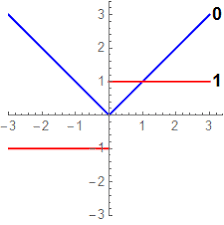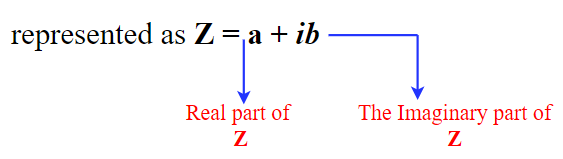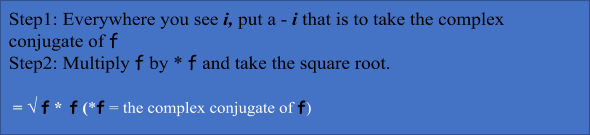Learn US

# Derivative, integral of absolute value function

0

 1 Introduction 2 Derivative of absolute value 3 Integral of Absolute Value 4 Magnitude of a complex number 5 Find the magnitude of a Complex Number 6 Summary 7 FAQs

1 October  2020

## Introduction

To understand the Absolute value of a Derivative and Integral or magnitude of a complex number

We must first understand what is the meaning of absolute value.

This would mean the absolute value of any number would always be positive irrespective of whether the given number is positive or negative.

E.g.: - Let’s say x is any positive number then the absolute value of x is |x|. Even if x is any negative number then also -x = |x|

We have another blog on absolute value function which deals with the function, Graphs, and Properties in detail.

## Derivative of absolute value

We can find the Derivative of an absolute value of any function with the help of the steps involving derivative of absolute value.

The formula for derivative of absolute value is defined as

d/dx |x| = (x* dy/dx) / |x|, where x will not be = 0Graph for Derivative of Absolute Value

We will understand this in detail with the below example: -

 Example 1

Find derivative of absolute value of x = |y-2|

Here x = |y-2|. Finding derivative of absolute value.

Now dx/ dy = d |y-2| / dy|y-2|So, dx/dy = [(y-2). (d(y-2)] / dy) / |y-2|

Hence dx/dy = (y-2). 1 / |y-2|

Therefore, dx/dy = (y-2) / |y-2|

Since denominator becomes 0 at y =2

Therefore, the derivative of absolute value dy/dx does not exist at y = 2.

Let's consider another example:

 Example 2

What is the derivative of absolute value function of y = |x2| and find the value of dy/dx at x = 2?

Here y = |x2

Therefore, by definition, dy /dx = (x2. d (x2) /dx) / |x2|

So dy/dx = (x2. 2x) / |x2|

Now at x = 2, dy/dx = (22 * 2(2)) / |22|

Therefore, at x = 2, dy/dx = (4*4)/ 4 = 4 which is the derivative of absolute value.

## Integral of Absolute Value

Please note that it's imperative to clearly understand what is Derivative of absolute value, and similarly Integration of Absolute value. Then I can assure you that the understanding of Integral of Absolute Value will be super easy and quick. But if not then please revisit the concept of the above 3 topics.

Let's perform Integral of Absolute value of x i. e.{ |x|  dx

So here we are going to break the above absolute value into pieces.

First, we know absolute value of |x| = X if X > 0 which is positive
Other end of absolute value of |x| = - X if X < 0 which is negative

So now integral of absolute value is
{ |x|  dx =  x dx (if X > 0 which is positive)
and {-x dx if X < 0 which is negative.

Or we can write Integral of absolute value of x is

{ |x|  dx   = 1/2x2 + c if X > 0 which is positive

And          = -1/2x2 + c if X < 0 which is negative

 Example

Find integral of absolute value of x.

\begin{align}\int\limits_0^6 {\left| {2x - 6} \right|dx} \end{align}

Now we know

$$\left| x \right| = \int {\begin{array}{*{20}{c}}{x,\rm{if}\,\,x > 0}\\{ - x,\rm{if}\,\,x < 0}\end{array}}$$

Therefore, with the above integral of absolute value of x,

2x-6 =            2x-6, If 2x-6 > 0, x > 3
-( 2x-6), If -2x+ 6 < 0, x < 3

So, we know when x is between 0 and 3 and then between 3 to 6. The integral of absolute value is

\begin{align}\int\limits_0^6 {\left| {2x - 6} \right|dx} \end{align}

\begin{align} = \int\limits_0^3 {\left| {( - 2x + 6} \right|dx + \int\limits_3^6 {\left| {( - 2x - 6} \right|dx} }\end{align}

Using the power Rule

-2x2/2 + 6x  3   + 2x2/2 - 6x     6
0                           3
-(3)2 + 6 (3) – 0 + (6)2 – 6 (6) – ((3)2 – 6 (3))

-9 + 18 - 0 + 36 – 36 – 9 + 18

= 18

## Magnitude of a complex number

The magnitude of a complex number or Absolute value of a complex number or Modulus of a complex number is the same thing.
But before we go into the details of the Magnitude of complex number lets quickly recap the basics of Complex number.

### Why do we need Complex numbers?

We all know that until now we were not able to take -ve of Real Numbers.

Let's understand this with the below equation.

X2 + 1 = 0
X2 = -1
X = ± √ -1             But, √ -1    does not exist in R

So, the answer to this equation is ± i

This √ -1   is denoted by the Symbol i which stands for iota.

Whenever we take the square root of - 1 i.e. i = √ -1 then an imaginary unit is introduced which does not belong to the real-world as of now. This is what is called iota.

The main form of Complex numbers isNow that we are comfortable with Complex numbers lets find out the magnitude of a complex number.

The magnitude of a Complex number is also called the magnitude of Imaginary numbers. What we are thinking about here is how big is the imaginary number. So, if we have any function or complex number
Let’s say for the below function we can calculate its magnitude of a complex number as

 Example 1

Find the absolute value of complex number. f = 2 i

To find the magnitude of a complex number we have to first follow below 2 important steps. Though it looks complicated, it is very simple.Here complex conjugate from the above function is

* f = 2(- i) = - 2 i

Therefore the magnitude of the above complex number is

|f| = √ f ×* f

= √ 2 i × - 2 i

= √ - 4 i2 ……………(a)

Now here we know that i = √ -1 therefore squaring both sides we get

i2= (√ -1)2 or i2 = -1

So, putting i2 = -1 in the above equation (a) we get

√ - 4 × (-1)    = √4 = 2

Therefore |f| = 2
So, 2 is the magnitude of 2 i.

## Find the magnitude of a Complex Number

Now here let’s take a complex number -3+5 i and plot it on a complex plane

In the above diagram, we have plot -3 on the Real axis and 4 on the imaginary axis. So, this complex is number -3+5 i is plotted right up there on the graph at point Z.

Now the absolute value of complex number is the distance from the point of origin (0,0) to point Z (-3,5) i.e. the point of a complex number.

We can now use the distance Formula where we plug in X coordinates of the two points and the Y coordinates of the two points.

\begin{align}&\sqrt {{{({x_2} - {x_1})}^2} + {{({y_2} - {y_1})}^2}} \\&= \sqrt {{{( - 3 - 0)}^2} + {{(5 - 0)}^2}} \\&= \sqrt {{{(3)}^2} + {{(5)}^2}} \text{or we can simply put}\sqrt {{a^2} + {b^2}} \\&= \sqrt {9 + 25} = \sqrt {34} = 5.8 \end{align}

(This is the absolute value of complex number -3+5 i )

## Summary

Here we have tried to understand we have gone through the basics of Derivative and Integration numbers along with understanding what and why the complex number is required. We also learned about the Magnitude of a Complex Number. However, it's always beneficial to understand the above concept with the help of a graph or diagram.

Yes

## How to find absolute value of complex numbers?Written by Rashi Murarka

GIVE YOUR CHILD THE CUEMATH EDGE
Access Personalised Math learning through interactive worksheets, gamified concepts and grade-wise courses